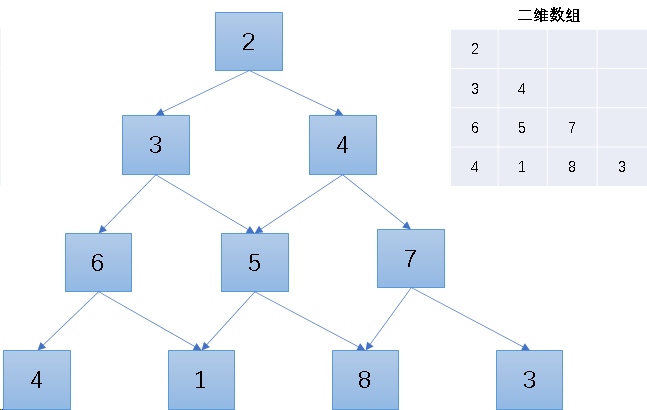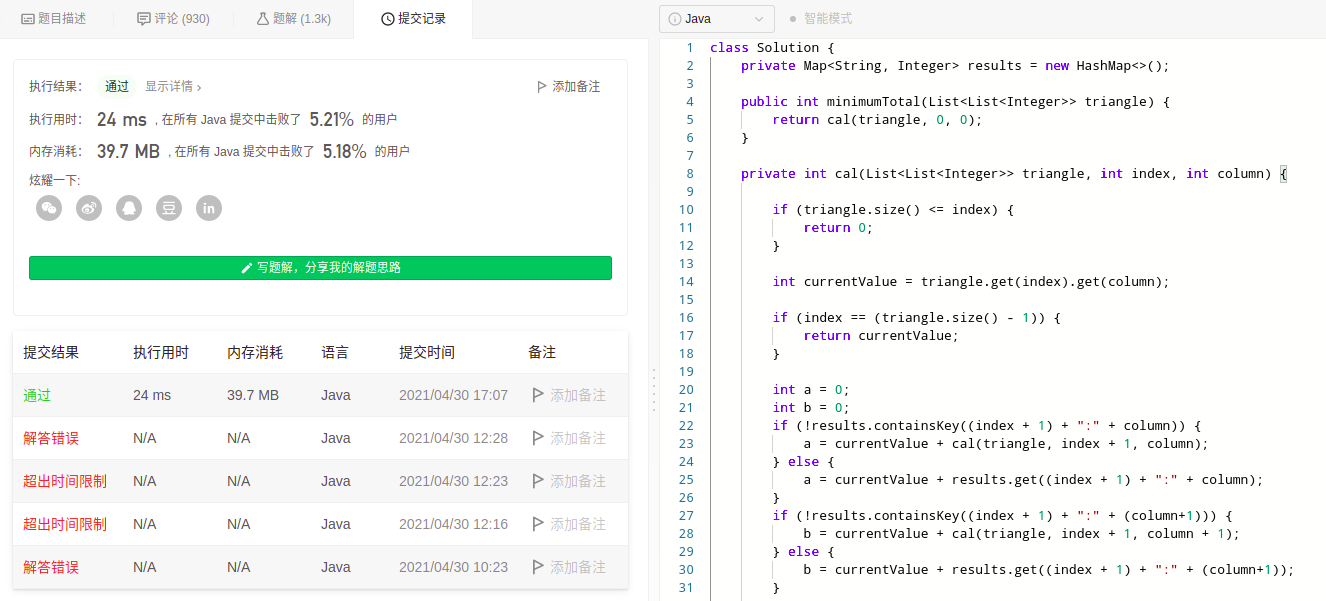## 题目描述

 输入：triangle = [,[3,4],[6,5,7],[4,1,8,3]] 输出：11 解释：如下面简图所示：    2   3 4  6 5 7 4 1 8 3 自顶向下的最小路径和为 11（即，2 + 3 + 5 + 1 = 11）。

 输入：triangle = [[-10]] 输出：-10

• 1 <= triangle.length <= 200
• triangle.length == 1
• triangle[i].length == triangle[i - 1].length + 1
• -104 <= triangle[i][j] <= 104

## 解题思路## AC代码

public class T120 {

// 存储计算结果
private Map<String, Integer> results = new HashMap<>();

public int minimumTotal(List<List<Integer>> triangle) {
return cal(triangle, 0, 0);
}

private int cal(List<List<Integer>> triangle, int index, int column) {

// 临界条件
if (triangle.size() <= index) {
return 0;
}

int currentValue = triangle.get(index).get(column);

if (index == (triangle.size() - 1)) {
return currentValue;
}

int a = 0;
int b = 0;

// 判断是否已经计算过
if (!results.containsKey((index + 1) + ":" + column)) {
// 递归调用(index+1, column)
a = currentValue + cal(triangle, index + 1, column);
} else {
a = currentValue + results.get((index + 1) + ":" + column);
}
// 判断是否已经计算过
if (!results.containsKey((index + 1) + ":" + (column+1))) {
// 递归调用(index+1, column)
b = currentValue + cal(triangle, index + 1, column + 1);
} else {
b = currentValue + results.get((index + 1) + ":" + (column+1));
}
// 比较，取最小值
if (a <= b) {
// 存入容器
results.put(index + ":" + column, a);
return a;
} else {
results.put(index + ":" + column, b);
return b;
}
}

@Test
public void test27() {

List<Integer> l1 = Arrays.asList(2);
List<Integer> l2 = Arrays.asList(3, 4);
List<Integer> l3 = Arrays.asList(6, 5, 7);
List<Integer> l4 = Arrays.asList(4, 1, 8, 3);
List<List<Integer>> input = new ArrayList<>();
int ret = minimumTotal(input);
System.out.println(ret);
}
}


## 总结